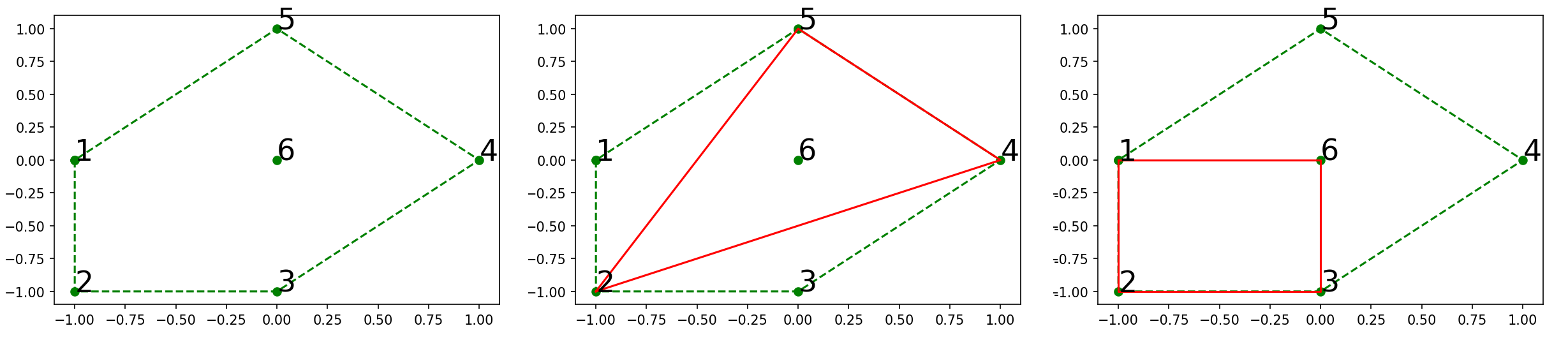# #319. 【NOI2017】分身术

"分！身！术！" —— 小P

"分！身！术！"

### 输入格式

$$p_i = [(S + c_i) ~\mathrm{mod}~ n] + 1$$

$$p_i = [(-1+c_i) ~\mathrm{mod}~ n] + 1$$

### 样例一

#### input

6 2
-1 0
-1 -1
0 -1
1 0
0 1
0 0
3 1 3 6
2 0 1



#### output

3
2



#### explanation### 限制与约定

11010$\leq n - 3$
210001000
3
4
5100000100000$=1$
6
7
8
9$=2$
10
11$\leq 3$
12$\leq 5$
13$\leq 9$
14$\leq 12$
15$\leq 20$
16$\leq 100$
17
18
19
20

• $|x_i|, |y_i|\leq 10^{8}$；
• 没有两个分身的坐标是完全相同的；
• $k \leq 100$；
• 所有时刻的 $k$ 之和不超过 $2 \times 10^{6}$；
• $0 \leq c_i \leq 2^{31}-1$；
• 初始时，所有的 $n$ 个分身占据区域面积大于 $0$；
• 定义所有 $n$ 个分身所占据区域的顶点集合为 $S$， $|S|\geq 3$。在任意时刻，$S$ 中至少存在两个未消失的分身。